# CBSE Class 7 Mathematics The Triangle And Its Properties Notes

Download CBSE Class 7 Mathematics The Triangle And Its Properties Notes in PDF format. All Revision notes for Class 7 Triangles and Its Properties have been designed as per the latest syllabus and updated chapters given in your textbook for Triangles and Its Properties in Standard 7. Our teachers have designed these concept notes for the benefit of Grade 7 students. You should use these chapter wise notes for revision on daily basis. These study notes can also be used for learning each chapter and its important and difficult topics or revision just before your exams to help you get better scores in upcoming examinations, You can also use Printable notes for Class 7 Triangles and Its Properties for faster revision of difficult topics and get higher rank. After reading these notes also refer to MCQ questions for Class 7 Triangles and Its Properties given our website

## Class 7 Triangles and Its Properties Revision Notes

Class 7 Triangles and Its Properties students should refer to the following concepts and notes for Triangles and Its Properties in standard 7. These exam notes for Grade 7 Triangles and Its Properties will be very useful for upcoming class tests and examinations and help you to score good marks

### Notes Class 7 Triangles and Its Properties

CBSE Class 7 The Triangle and its Properties Concepts. Learning the important concepts is very important for every student to get better marks in examinations. The concepts should be clear which will help in faster learning. The attached concepts made as per NCERT and CBSE pattern will help the student to understand the chapter and score better marks in the examinations.

The Triangle and its Properties

Triangle

triangle is a closed figure made of three line segments. Every triangle has three sides, three angles, and three vertices. These are known as the parts of a triangle. The sides and the angles of every triangle may differ from one another; therefore, they do not look alike.

Classification of Triangles

Triangles can be classified based on their sides and angles.

• Based on their sides, there are equilateralisosceles and scalene triangles.
• Based on their angles, there are acuteobtuse and right-angled triangles.

Equilateral triangle: A triangle in which all the sides are equal is called an equilateral triangle. All the three angles of an equilateral triangle are also equal, and each measures 60°.

Isosceles triangle: A triangle in which any two sides are equal is called an isosceles triangle. In an isosceles triangle, the angles opposite the equal sides are called the base angles, and they are also equal.

Scalene triangle: A triangle in which no two sides are equal is called a Scalene triangle.

Acute-angled triangle: A triangle with all its angles less than 90° is known as an acute-angled triangle.

Obtuse-angled triangle: A triangle with one of its angles more than 90° and less than 180° is known as an obtuse-angled triangle.

Right-angled triangle: A triangle with one of its angles equal to 90° is known as a right-angled triangle. The side opposite the 90° angle is called the hypotenuse, and is the longest side of the triangle.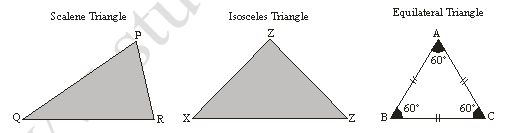Median and Centroid of a triangle

Mark the mid-point of the side of a triangle, and join it to its opposite vertex. This line segment is called a median. It is defined as a line segment drawn from a vertex to the mid-point of the opposite side.  Three medians can be drawn  to a given triangle. The medians pass through a common point. Hence, the medians of a triangle are concurrent. This point of concurrence is called the centroid, and is denoted by G. The centroid and medians of a triangle always lie inside the triangle. The centroid of a triangle divides the median in the ratio 2:1.

The three medians of a triangle meet at one point inside the triangle. This point is called the
centroid.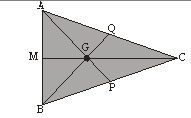Altitude and Orthocentre

The altitude of a triangle is a line segment drawn from a vertex and is perpendicular to the opposite side. A triangle has three altitudes. The altitudes of a triangle are concurrent. The point of concurrence is called the orthocentre, and is denoted by O. The altitude and orthocentre of a triangle need not lie inside the triangle.

Three altitudes of a triangle always intersect at one point, called the orthocentre.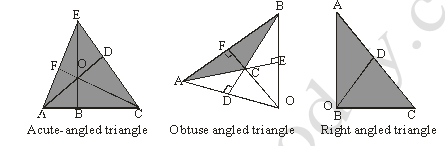Properties of Triangle

Angle sum property:

The sum of the three angles in a triangle is equal to 180°

Eg:  If A, B and C are the angles of a triangle, then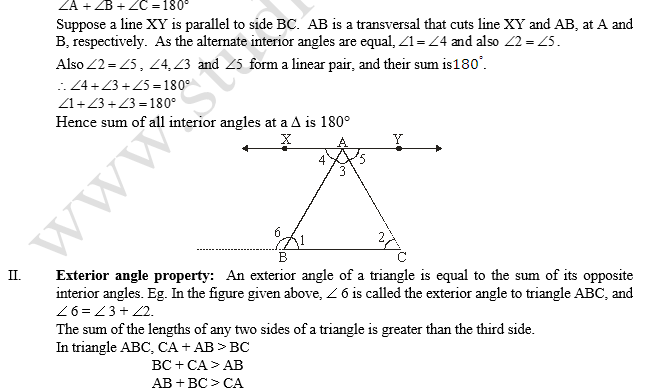Right Angled Triangle: In a right-angled triangle, the side opposite the right angle is called the hypotenuse, and the other two sides are called its legs.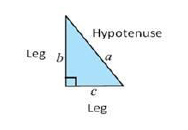III. Pythagorean theorem:

In a right-angled triangle, the square of the hypotenuse is equal to the sum of the squares of the other two legs. i.e. a2 = b2 + c2

Converse of Pythagorean Theorem

If the Pythagoras property holds, then the triangle must be right-angled. That is, if there is a triangle such that the sum of the squares on two of its sides is equal to the square of the third side, then it must be a right-angled triangle. The angle opposite to the third side is right angle.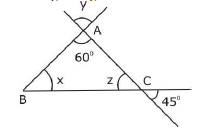Solution: In the given figure,

y = 60°(vertically opposite angles)

z = 45°(vertically opposite angles ) In triangle ABC

∠A + ∠B + ∠C

= 180 ° (By angle sum property of triangle)

⇒ 60°+ x + 45°= 180°

⇒ x + 105°= 180°

⇒ x = 180°– 105°

⇒ x = 75°

Example 2: Find angles and in the following figure.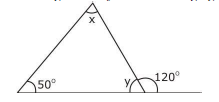Solution: In the given figure,

∠y + 120° = 180° (since they are linear pair)

⇒ ∠y = 180° - 120° = 60°

Also ∠x + 50° = 120° (by exterior angle property of triangle)

⇒ ∠x = 120° - 50°

⇒ ∠x = 70°

∴ ∠x = 70° and ∠y = 60°

Example 3: Find angle in the following figure: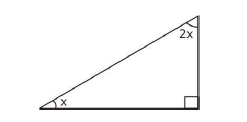Solution: Sum of the angles of a triangle = 180°

2x + x + 90° = 180°

⇒ 3x = 90°

⇒ x = 30°

Example 4: A 15 m long ladder reached a window 12 m high from the ground on placing it against a wall at a distance ‘a’ metre from the wall. Find the distance of the foot of the ladder from the wall.

Solution: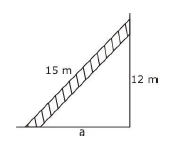By using Pythagoras theorem, We have

⇒ a2 + 122 = 152 ⇒ a2 = 225 – 144

⇒ a2 = 81 ⇒ a2 = 92

⇒ a = 9m

Thus the ladder is 9 m away from the foot of the wall.

Example 5: If in a right angle isosceles triangle area is 32 cm2 . Find the sides of the triangle.

Solution: Area of triangle = 1/2 (base × altitude)

Base = Altitude = x

1/2( x * ) = 32 ⇒ x2 = 64

x = 8 cm

Therefore two sides containing right angle are equal to 8 cm

Third side of triangle = √(82 + 82)= 8√2 cm

Example 6: Find the perimeter of a rectangle whose length is 40 cm and the length of one of the diagonal is 41 cm.

Solution: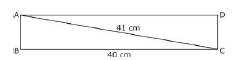Now in DABC, AB ⊥ BC

∴ AB2 + BC2 = AC2

⇒  AB2 + 402 = 412 ⇒ AB2 = 1681 – 1600

⇒ AB2 = 81 ⇒ AB2 = 92 or AB = 9

∴ Perimeter = 2 (AB + BC) = 2(9 + 40)

= 2 × 49 = 98 cm

Example 7: Can 5cm, 7cm and 5cm be the sides of a triangle?

Solution: Check whether the sum of any two sides is greater than the third side or not:

5+ 7 =12 cm > 5 cm.

7+5 =12 cm > 5 cm

5 + 5 = 10 cm > 7 cm

Therefore, these measurements can be the sides of a triangle.

## Tags:

Click for more Triangles and Its Properties Study Material
 CBSE Class 7 Mathematics The Triangle And Its Properties Notes

## Latest NCERT & CBSE News

Read the latest news and announcements from NCERT and CBSE below. Important updates relating to your studies which will help you to keep yourself updated with latest happenings in school level education. Keep yourself updated with all latest news and also read articles from teachers which will help you to improve your studies, increase motivation level and promote faster learning

### Pariksha Pe Charcha 2022

The 5th edition of Pariskhas Pe Charcha the unique interactive program of Hon’ble Prime Minister with students teaches and parents will be held through virtual mode in February, 2022. In order to select participants who will be featured in Pariksha Pe Charcha programme...

This is with reference to CBSE Notification No. 40/2021 dated 04.05.2021 regarding the Innovation Ambassador program – An online training program for teachers by CBSE in collaboration with Ministry of Education’s Innovation Cell (MIC) and AICTE. In view of the current...

### Celebration of Matribhasha Diwas Mother Language day

UNESCO has declared 21st February of every year to be celebrated as International Mother Language day to promote dissemination of Mother Language of all, create awareness of linguistic and cultural traditions and diversity across the world and to inspire solidarity...

### CBSE Term 2 Board Examinations

CBSE vide Circular No.Acad-51/2021 dated 5th July, 2021, notified that in the session 2021-2022, Board Examinations would be conducted in two terms, i.e.. Term I and Term II. This decision was taken due to the uncertainty arising out of COVID 19 Pandemic. Term I...

### CBSE Science Challenge 2021 22

Science is inexplicably linked with our lives and helps us to understand the world around us better. Scientific and technological developments contribute to progress and help improve our standards of living. By engaging with this subject, students learn to think, solve...

### Surya Namaskar Project on 75th Anniversary of Independence Day

Ministry of Education, Govt of India vide letter No. F.No. 12-5/2020-IS-4 dated 16.12.2021 has intimated that under the banner Azadi ka Amrit Mahotsav the National Yogasanasports Federation has decided to run a project of 750 million Surya Namaskar from 01 January 2022...